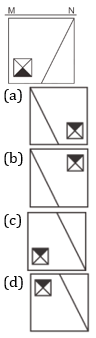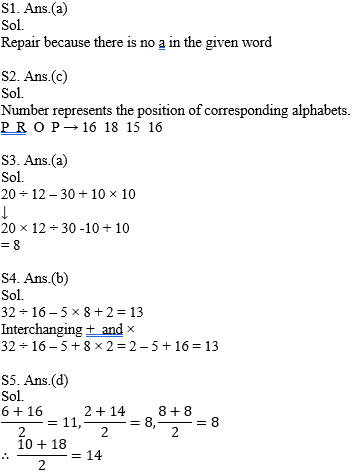The reasoning is the most scoring section in any competitive exam. It also develops or nourishes our critical thinking skills as compared to other subjects. In DSSSBKVSNVS Exam, as a student to score well in this part, you have to start your preparation now because there is negative marking as well. The more you have prepared, the better you score.

Q1. From the given alternatives, select the word which CANNOT be formed using the letters of the given word.: Represhensible

(a) Repair
(b) Hen
(c) Rise
(d) Ripe

Q2. In a certain code language, “FUMIC” is written as “6211393” and “STEAM” is written as “19205113”. How is “PROP” written in that code language?

(a) 714812
(b) 6159134
(c) 16181516
(d) 1271418

Q3. In a certain code language, 'x' represents '+', '÷' represents 'x', '-' represents '÷' and '+' represents '-'. Find out the answer to the following question.
20 ÷ 12 - 30 + 10 x 10 = ?

(a) 8
(b) 25
(c) 14
(d) 21

Q4. The following equation is incorrect. Which two signs should be interchanged to correct the equation?
32 ÷ 16 - 5 x 8 + 2 = 13

(a) + and ÷
(b) + and x
(c) - and +
(d) ÷ and x

Q5. If 6@16 = 11, 2@14 = 8 and 8@8 = 8, then ﬁnd the value of 10@18 = ?

(a) 4
(b) 6
(c) 12
(d) 14

Q6. A series is given with one term missing. Select the correct alternative from the given ones that will complete the series.
API, CRK, ETM, ?, IXQ

(a) HUP
(b) GVO
(c) IVP
(d) IUO

Q7. In the following question, select the missing number from the given series.
108, 104, 100, 96, 92, ?

(a) 88
(b) 84
(c) 90
(d) 86

Q8. In the following question, four groups of three numbers are given. In each group the second and third number are related to the first number by a Logic/Rule/Relation. Three are similar on basis of same Logic/Rule/Relation. Select the odd one out from the given alternatives.

(a) (14, 29, 43)
(b) (15, 31, 46)
(c) (18, 37, 52)
(d) (13, 27, 40)

Q9. If a mirror is placed on the line MN, then which of the answer figures is the right images of the given figure?Q10. Which of the following cube in the answer figure cannot be made based on the unfolded cube in the question figure?Solutions